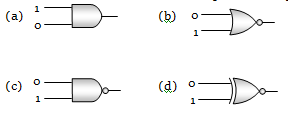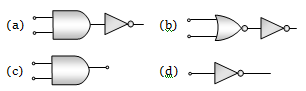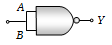Boolean algebra is essentially based on

(a) Truth                     (b) Logic
(c) Symbol                  (d) Numbers

Concept Questions :-

Logic gates
High Yielding Test Series + Question Bank - NEET 2020

Difficulty Level:

The logic behind ‘NOR’ gate is that it gives

(a) High output when both the inputs are low

(b) Low output when both the inputs are low

(c) High output when both the inputs are high

(d) None of these

Concept Questions :-

Logic gates
High Yielding Test Series + Question Bank - NEET 2020

Difficulty Level:

A logic gate is an electronic circuit which

(a) Makes logic decisions

(b) Allows electrons flow only in one direction

(c) Works binary algebra

(d) Alternates between 0 and 1 values

Concept Questions :-

Logic gates
High Yielding Test Series + Question Bank - NEET 2020

Difficulty Level:

A gate has the following truth table
P 1 1 0 0
Q 1 0 1 0
R 1 0 0 0

The gate is
(a) NOR                      (b) OR
(c) NAND                    (d) AND

Concept Questions :-

Logic gates
High Yielding Test Series + Question Bank - NEET 2020

Difficulty Level:

Which of the following gates will have an output of 1Concept Questions :-

Logic gates
High Yielding Test Series + Question Bank - NEET 2020

Difficulty Level:

Which represents NAND gateConcept Questions :-

Logic gates
High Yielding Test Series + Question Bank - NEET 2020

Difficulty Level:

What will be the input of A and B for the Boolean expression $\overline{\left(\mathrm{A}+\mathrm{B}\right)}·\left(\overline{\mathrm{A}·\mathrm{B}}\right)=1$
(a) 0, 0                            (b) 0, 1
(c) 1, 0                            (d) 1, 1

Concept Questions :-

Logic gates
High Yielding Test Series + Question Bank - NEET 2020

Difficulty Level:

If A and B are two inputs in AND gate, then AND gate has an output of 1 when the values of A and B are
(a) A = 0, B = 0                         (b) A = 1, B = 1
(c) A = 1, B = 0                         (d) A = 0, B = 1

Concept Questions :-

Logic gates
High Yielding Test Series + Question Bank - NEET 2020

Difficulty Level:

The Boolean equation of NOR gate is
(a) C = A + B               (b) $\mathrm{C}=\overline{\mathrm{A}+\mathrm{B}}$
(c) $C=A·B$                 (d) $\mathrm{C}=\overline{\mathrm{A}.\mathrm{B}}$

Concept Questions :-

Logic gates
High Yielding Test Series + Question Bank - NEET 2020

Difficulty Level:

This symbol represents(a) NAND gate
(b) OR gate
(c) AND gate
(d) NOR gate

Concept Questions :-

Logic gates